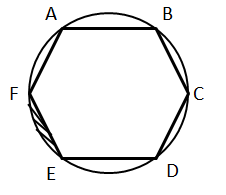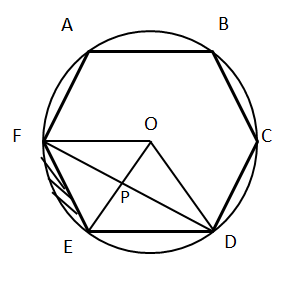# CAT Quantitative Aptitude Questions | Geometry Questions for CAT - Triangles

###### CAT Questions | CAT Geometry Questions | Regular Polygon

The question is from regular polygon. It discusses about a regular hexagon inscribed inside a circle. CAT Geometry questions are heavily tested in CAT exam. Make sure you master Geometry problems. Triangles are heavily tested, the wonderful infinite-sided polygon that is the circle is also heavily tested. In between these two lies this great mass of regular polygons.

Question 13: ABCDEF is a regular hexagon inscribed inside a circle. If the shortest diagonal of the hexagon is of length 3 units, what is the area of the shaded region.1. 1/6(3π − (9√3)/2)
2. 1/6(2π − (6√3)/2)
3. 1/6(3π − (8√3)/2)
4. 1/6(6π − (15√3)/2)

## Best CAT Coaching in Chennai

#### CAT Coaching in Chennai - CAT 2021Online Batches Available Now!

##### Method of solving this CAT Question from Regular Hexagon: A regular hexagon is just 6 equilateral triangles around a point.Let side of regular hexagon be a.
The shortest diagonal will be of length a√3. Why?
A regular hexagon is just 6 equilateral triangles around a point. The shortest diagonal is FD.
FD = FP + PD
△FOE is equilateral and so is △ EOD.
Diagonal FD can be broken as FP + PD, both of which are altitude of equilateral �?�s.
FP = (√3a)/2
FD = √3a = shortest diagonal
The question tells us that the shortest diagonal measures 3 cm.
√3a = 3 => a = √3
Area of hexagon = (√3a2 )/4 * 6
Area of circle – area of hexagon = π (√3)2 − √3/4 * (√3)2 * 6
= 3π − (9√3)/2
Area of shaded region = 1/(6 ) (area(circle) – area(hexagon))
= 1/(6 )(3π − (9√3)/2)

The question is "what is the area of the shaded region?"

##### Hence, the answer is 1/(6 )(3π − (9√3)/2)

Choice A is the correct answer.

###### CAT Coaching in ChennaiCAT 2021Enroll at 49,000/- 44,000/-

Online Classroom Batches Starting Now!

###### Best CAT Coaching in ChennaiPrices slashed by Rs 4000/-

Attend a Demo Class

## CAT Preparation Online | CAT Geometry questions Videos On YouTube

#### Other useful sources for Geometry Question | Geometry Triangles Circles Quadrilaterals Sample Questions

##### Where is 2IIM located?

2IIM Online CAT Coaching
A Fermat Education Initiative,
58/16, Indira Gandhi Street,
Kaveri Rangan Nagar, Saligramam, Chennai 600 093

##### How to reach 2IIM?

Phone: (91) 44 4505 8484
Mobile: (91) 99626 48484
WhatsApp: WhatsApp Now
Email: prep@2iim.com